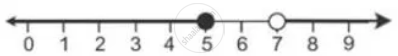Share

# Solve and Graph the Solution Set Of: 3x – 2 > 19 Or 3 – 2x ≥ -7, X ∈ R - Mathematics

Course

#### Question

Solve and graph the solution set of:

3x – 2 > 19 or 3 – 2x ≥ -7, x ∈ R

#### Solution

3x – 2 > 19 or 3 – 2x ≥ -7

3x > 21 or -2x ≥ -10

x > 7 or x ≤ 5

Graph of solution set of x > 7 or x ≤ 5 = Graph of points which belong to x > 7 or x ≤ 5 or both.

Thus, the graph of the solution set is:Is there an error in this question or solution?